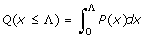P(x) λe -λxQ(x ≤ Λ) 1 - e-λΛ1. We have used five different probability distribution functions to fit the numerically obtained effects of mutations on fitness. We show the functional form of the probability distribution (PDF) P(x) and the corresponding cumulative probability distribution (CPF),for each case. In our fits, we have used the latter, with parameters that yield the minimum least-squares deviation from our numerical results.